# Deep Deterministic Policy Gradient (DDPG) (Tensorflow)

## 算法 ¶

DDPG 的算法实际上就是一种 Actor Critic, 我在上一篇中简短地介绍了 Actor Critic 的算法. 不太清楚的同学先去看看上一篇吧.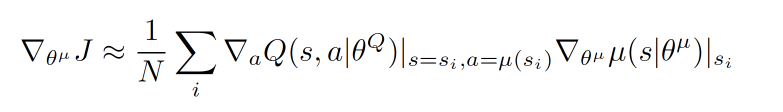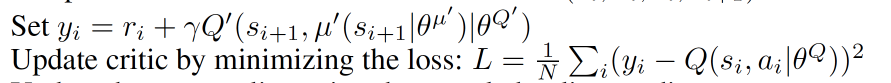## 主结构 ¶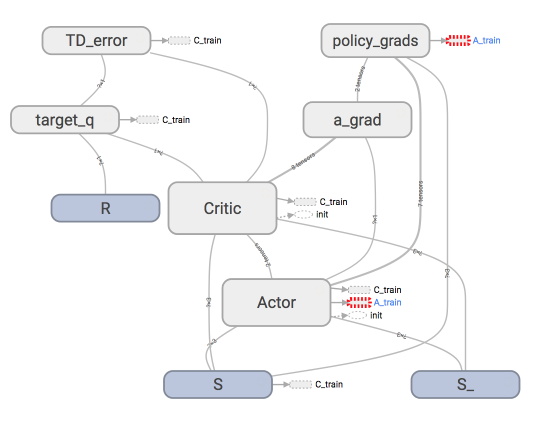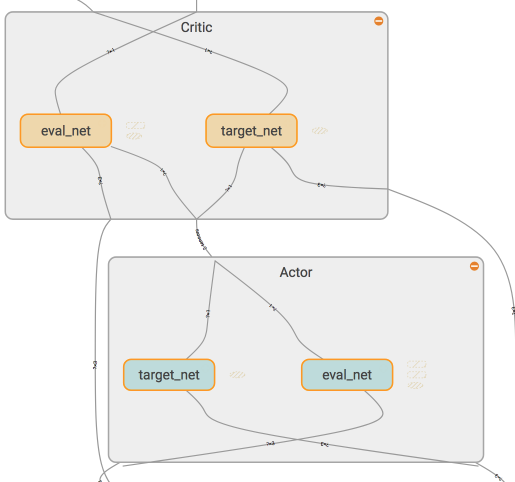class Actor(object):
def __init__(self):
...
with tf.variable_scope('Actor'):
# 这个网络用于及时更新参数
self.a = self._build_net(S, scope='eval_net', trainable=True)
# 这个网络不及时更新参数, 用于预测 Critic 的 Q_target 中的 action
self.a_ = self._build_net(S_, scope='target_net', trainable=False)
...

class Critic(object):
def __init__(self):
with tf.variable_scope('Critic'):
# 这个网络是用于及时更新参数
self.a = a  # 这个 a 是来自 Actor 的, 但是 self.a 在更新 Critic 的时候是之前选择的 a 而不是来自 Actor 的 a.
self.q = self._build_net(S, self.a, 'eval_net', trainable=True)
# 这个网络不及时更新参数, 用于给出 Actor 更新参数时的 Gradient ascent 强度
self.q_ = self._build_net(S_, a_, 'target_net', trainable=False)


## Actor Critic ¶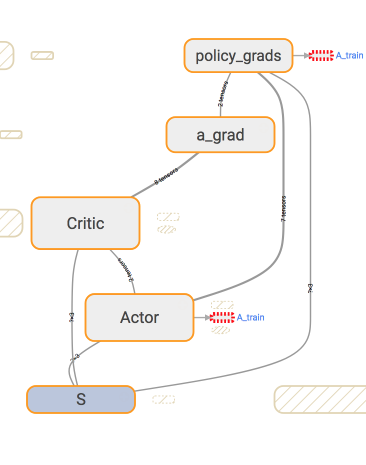with tf.variable_scope('policy_grads'):
# 这是在计算 (dQ/da) * (da/dparams)
ys=self.a, xs=self.e_params, # 计算 ys 对于 xs 的梯度
)
with tf.variable_scope('A_train'):


with tf.variable_scope('a_grad'):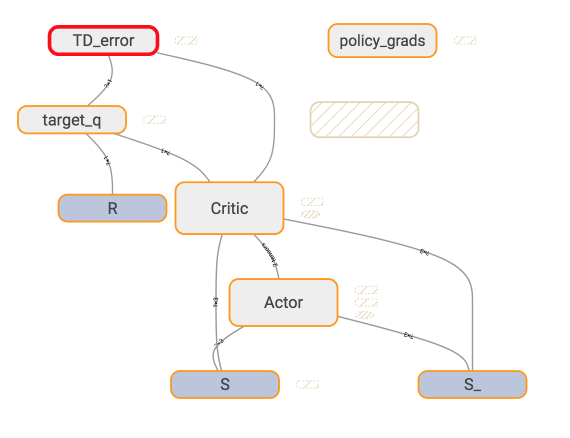# 计算 target Q
with tf.variable_scope('target_q'):
self.target_q = R + self.gamma * self.q_    # self.q_ 根据 Actor 的 target_net 来的
# 计算误差并反向传递误差
with tf.variable_scope('TD_error'):
self.loss = tf.reduce_mean(tf.squared_difference(self.target_q, self.q))  # self.q 又基于 Actor 的 target_net
with tf.variable_scope('C_train'):


actor = Actor(...)
critic = Critic(..., actor.a, actor.a_)  # 将 actor 同它的 eval_net/target_net 产生的 a/a_ 传给 Critic


## 记忆库 Memory ¶

class Memory(object):
def __init__(self, capacity, dims):
"""用 numpy 初始化记忆库"""

def store_transition(self, s, a, r, s_):
"""保存每次记忆在 numpy array 里"""

def sample(self, n):
"""随即从记忆库中抽取 n 个记忆进行学习"""


## 每回合算法 ¶

var = 3  # 这里初始化一个方差用于增强 actor 的探索性

for i in range(MAX_EPISODES):
...
for j in range(MAX_EP_STEPS):
...

a = actor.choose_action(s)
a = np.clip(np.random.normal(a, var), -2, 2) # 增强探索性
s_, r, done, info = env.step(a)

M.store_transition(s, a, r / 10, s_)   # 记忆库

if M.pointer > MEMORY_CAPACITY: # 记忆库头一次满了以后
var *= .9998    # 逐渐降低探索性
b_M = M.sample(BATCH_SIZE)
...   # 将 b_M 拆分成下面的输入信息
critic.learn(b_s, b_a, b_r, b_s_)
actor.learn(b_s)

s = s_

if j == MAX_EP_STEPS-1:
break